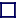### PACKING FRACTION (PF)

 The mass of an isotope is not simply the sum of the masses of all the constituent nucleons (protons and neutrons) within the nucleus, because when they are all gathered together inside the nucleus, some of the mass is 'lost' this loss being called the 'mass defect', being the atomic mass, m minus the mass number, A. MD=(m-A), where A is the number of protons + neutrons. The packing fraction, or mass defect per nucleon, is numerically equal to the mass defect divided by the mass number, A. PF=(m-A)/A. The packing fraction is zero for 12C (by definition) and has a minimum value at about Z=26, iron., where Z is the number of protons. The above chart shows the packing fractions for the known isotopes of Barium. note that they are lowest near the valley of stability (the beta/inverse beta watershed).